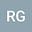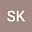loading page

Chebyshev collocation method for the variable-order fractional diffusion equation with a variable diffusion coefficient
••## Abstract

In this paper, we study the space-time variable-order fractional diffusion equation with a variable diffusion coefficient. The fractional derivatives of variable-orders are considered in the Caputo sense. We propose a numerically efficient pseudospectral method with Chebyshev polynomial as an orthogonal basis function. Also, we examine the error analysis of the given numerical approach. A variation on the maximum absolute error with the different variable orders in space and time are studied. Some illustrative examples are presented with different boundary conditions, e.g., Dirichlet, mixed, and non-local. The applicability of the method is also tested with the problem that has fractional power in solution. The results obtained from the proposed method prove the efficacy and reliability of the method.